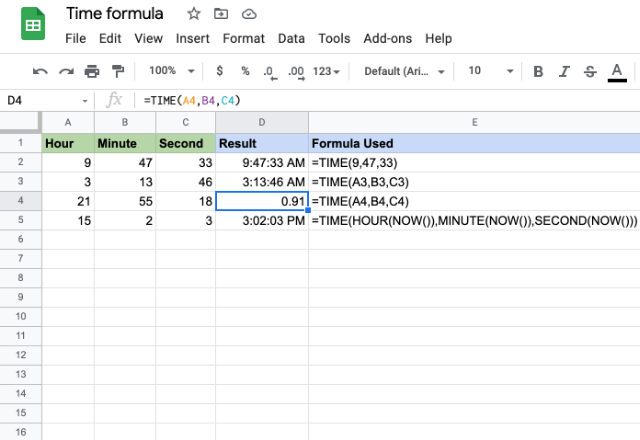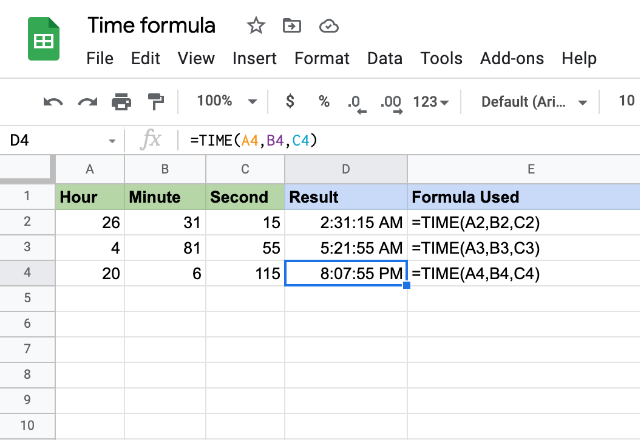# How to use the TIME formula in Google SheetsThe TIME formula in Google Sheets accepts a given time through the hour, minute, and second component values, and returns a time type value.

### Syntax

TIME(hour, minute, second)

The hour, minute and second are self-explanatory parameters that accept corresponding components of a time. These can be direct numeric values or references to cells that have corresponding numeric values. Also, the respective parameter inputs can be outputs that the formulas such as HOUR, MINUTE and SECOND returns.

### Usage: TIME formula in Google Sheets

Using this formula is a pretty straightforward process. Let us understand the formula using a few examples.Please notice that all the outputs are ‘time’ formatted, except the third, which ‘number’ formatted. This is done deliberately, just to illustrate that a time is a number after all. You may recall that a date is a natural number. However, the time is a decimal number.

The first example accepts direct numbers and returns a corresponding time value. The parameters for the second and third examples are references to cells that contain hour, minute and second values. Accordingly, we get the output times.

The final example shows that the TIME formula in Google Sheets can also accept other appropriate formulas for its parameters. And, it returns yet another time as expected.

#### Note

What happens if we try and input a value that is not valid in reality? Please have a look at the following image.In each of these examples, you will notice we have tried to input invalid hour, minute and second values respectively. Yet, we don’t see any errors in the output values. That is because the TIME formula is capable of appropriately offsetting the excess values from the hour, minute or second parameters.

For instance, in the first example, 26 hours correspond to 1 day + 2 hours, wherein it disregards the day component and considers only 2 hours for its hourcomponent. Similarly, 81 minutes in the second example is equivalent to 1 hour + 21 minutes. So, it adds the extra 1 hour is to the hour component and considers 21 minutes for the minute component. On the similar lines, 115 seconds in the third example is equal to 1 minute + 55 seconds. Accordingly, the TIME formula increments the minute component by 1 minute, while it picks up 55 seconds for the second component.

### TIME formula

And there you go! Use the TIME formula in Google Sheets to return a time type value.